# Lecture 2 - bancron/stanford-cs224n Wiki

This lecture covers more about word vectors, word senses, and neural network classifiers.

## More about gradient descent to calculate/train word vectors

Reminder: we start with random word vectors. Take a large corpus. For each word, predict what words surround the center word using a probability distribution derived from the dot product between the center word and the context words. We "learn" by updating the word vectors so they predict the context words better.

Doing no more than this simple algorithm, this allows us to learn word vectors that well-capture word similarity in a word space. This is a bag of words model - a model that pays no attention to word order or position when estimating probability. This is fairly crude but still allows us to learn a lot about the properties of words.

We want a model that gives a reasonably high probability estimate to all words that occur in the context (at all often). Word2vec does this by placing words that are similar close to one another in the high-dimensional space. In a high-dimensional space, vectors can be close to many other vectors but close to them along different dimensions.

We calculate this via gradient descent. The idea is that from the current value of theta, we calculate the gradient J(theta), then take a small step in the direction of the negative gradient, updating theta. Then repeat. If you use a tiny step size, it make take a very long time to calculate the function. If the step size is much too big, you can diverge and start going to worse places. Our objectives are often not convex, but it generally turns out okay anyway.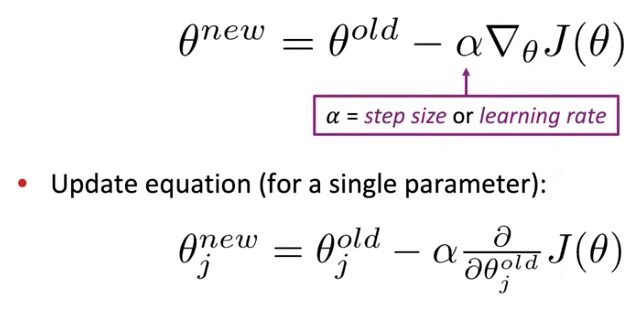This is the very simple gradient descent algorithm. Nobody uses it, and you should not use it either.

The problem is that J(theta) is a function of all windows in the corpus (often billions of words), so calculating the gradient of J(theta) is very expensive to compute. This is a bad idea for pretty much all neural nets.

## Stochastic gradient descent

Instead we will use stochastic gradient descent. We take a small batch (e.g. 32) center words, calculate an estimate of the gradient based on them, and use it to update your theta parameters. Now we can make a billion updates to the parameters as we pass through the corpus once, rather than only a single (but more accurate) update per pass through the corpus. We will use this algorithm everywhere.

It turns out this is not only a performance hack. The fact that stochastic gradient descent bounces around makes us learn better solutions that running the non-stochastic algorithm.

In each window, we have only at most 2m + 1 words (e.g. m = 5), so the gradient of J(theta) is very sparse.

"I like learning" -> `[0 0 0 ... 0 0 0 like 0 0 0 ... 0 0 0 I 0 0 0 ... 0 0 0 learning 0 0 0 ...]`

We might update only the word vectors that actually appear. We need either sparse matrix update operations to only update certain rows. (Note: mathematically these should be column vectors, but in all common deep learning packages including pytorch, word vectors are represented as row vectors. This is for efficiency - you can access an entire word vector as a single contiguous memory region.)

## Model variants

Use a single vector for a word, rather than two vectors averaged at the end. This is more complex to calculate because if the center word and context word are the same word, you have a messy case with a dot product `x . x`.

Skip-grams (SG) is the model we've been looking at - predict the context ("outside") words (position independent) given the center word.

Continuous Bag of Words (CBOW) predicts the center word from a bag of context words.

So far we've looked at naive softmax, which is simpler but more expensive to calculate. Particularly, calculating the denominator is quite expensive. Another option is negative sampling. We will train binary logistic regression models for both the true pair (the center word and a word in its context window) versus several (maybe 10-15) noise pairs (the center word paired with a random word in the vocabulary).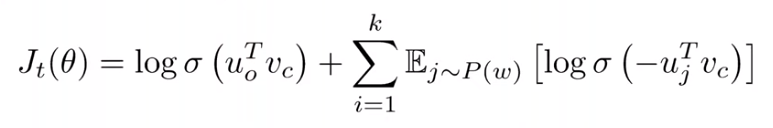sigma(x) is the sigmoid function. We use the negated dot product for the j negative samples. We maximize the probability that the real outside word appears and minimize the probability that random words appear around the center word. We have these extra negative samples to make the denominator larger - dot products with a word in the context large, dot products of words not in the context large, but we don't want to invent data.

We also sample with P(w) = U(w)^(3/4)/Z. U(w) is the unigram distribution of words in the corpus (i.e. normalized count). This power makes less frequent words be sampled more often as compared to a uniform distribution over the vocabulary.

## Co-occurrence vectors

Another idea: build a co-occurrence matrix of counts. Toy example with window size 1: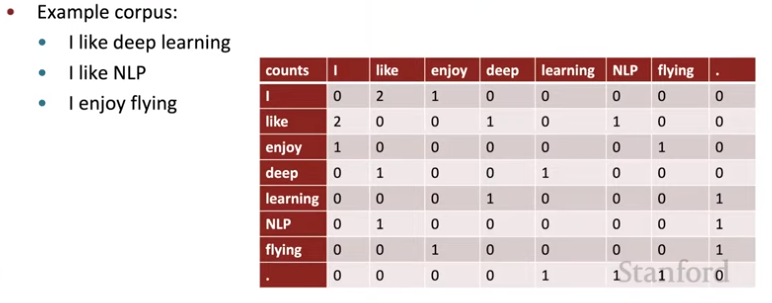Representation of "I" is the first row vector. We might expect "you" to have a similar vector. You could either use a window around the word, or use the full document, where a document could be a web page or a paragraph.

For simple count co-occurrence vectors, they are very high-dimensional with a large vocabulary. They require a lot of storage, and have sparsity issues, leading to less robust models.

We could use low-dimensional vectors (maybe 25-1000 dimensions, similar to Word2vec). How should we reduce the dimensionality?

Singular Value Decomposition of co-occurrence matrix X. De-compose into U, Sigma, and V^T.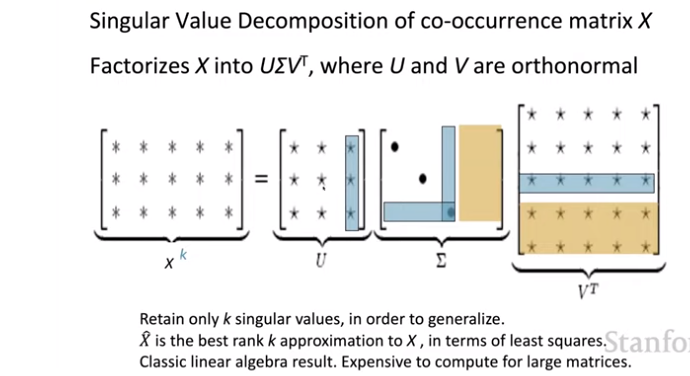The yellow parts are ignored (never used). We can further delete out some usually-low values from U and V (blue).

Unfortunately this works poorly because we would want normally distributed errors, and these word counts are not normally distributed at all - very high counts for "the", etc. and a long tail of low counts.

What if we scale the counts in the cells?

1. take log of the frequencies
2. cap values at e.g. 100
3. stop words list
4. ramped windows that count further-away words less

This works, roughly parallel to Word2vec.

## GloVe

GloVe algorithm - GloVe: Global Vectors for Word Representation (2014), co-authored by Chris Manning (our lecturer). Combine linear algebra methods with Skip-gram/CBOW.

Linear algebra methods train quickly and use statistics efficiently (meaning doing statistics on the entire vector at once to minimize loss), but primarily are used to capture word similarities, and give disproportionate importance to large counts. The models scale with corpus size (trading time for space). They make inefficient use of statistics, but have improved performance on other tasks, including complex patterns beyond word similarity (e.g. analogy task).

We want meaning components (e.g. queen->king, truck->driver) to be represented as ratios of co-occurrence probabilities.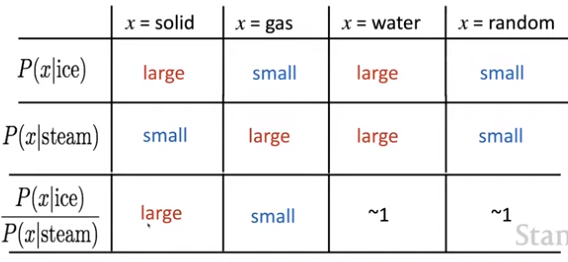(actual):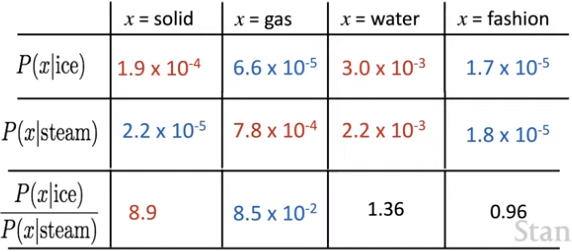How can we capture ratios of co-occurrence probabilities as linear meaning components in a word vector space? We can use a log-bilinear model with vector differences. We want the dot product to represent the log-probability of co-occurrence - so it's linear in each input (w[i], w[j]), which is related to the log of the probability.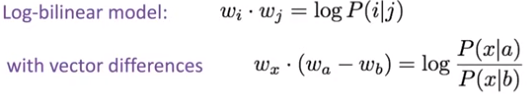We want this squared term to be as small as possible. There are also some bias terms (one for each word) to move things up and down for the word in general.

The GloVe model tries to unify the co-occurrence model and the neural model by training a neural model on top of a co-occurrence count.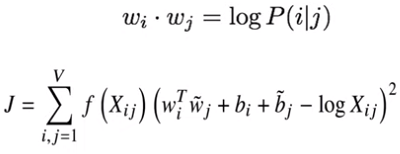We use an f function to scale things depending on the frequency of a word. We want to consider a high co-occurrence count, but for extremely common words (like function words) we cap how much that affects their similarity.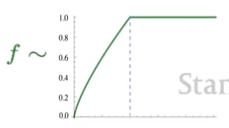Then we optimize the J function directly on the co-occurrence matrix. This works pretty well. But how do we evaluate word vectors?

## Evaluation metrics

We have intrinsic vs extrinsic measures (general NLP terms).

• Intrinsic evaluations
• evaluate directly on a specific/intermediate subtask
• they are fast to compute
• they help you understand the component you've been developing
• it's not clear that this generalizes to the problem you're trying to solve
• Extrinsic evaluations
• end-to-end evaluation on a real task
• they can take a long time to compute
• it's not clear if your subsystem is the issue, or if it's the integration with other subsystems
• if replacing exactly one subsystem with another improves accuracy, that's a clear sign of improvement

Example intrinsic word vector evaluation: get a large set of analogies. Evaluate the word vectors by how well their cosine distance after addition captures intuitive semantic and syntactic analogy questions. Discard the input words from the search. The Word2vec people build a large set of analogies, semantic (man : woman :: king : ?) and syntactic (short : shorter : shortest :: slow : ? : ?).

Why did GloVe do well? Not just the neural algorithm. It's important to have good data. More is better; Wikipedia is better than news text. (Word2vec was trained only on Google news wire data.) We also want an appropriate dimensionality - around 300 is a sweet spot.

Another intrinsic evaluation: how closely do they model human judgments of word similarity? Luckily psychologists have been asking people for judgments of similarity of words for several decades (e.g. scale of 0-10). Measure a correlation coefficient - do they give the same order of similarity judgments? Again GloVe did well.

Extrinsic word vector evaluation: Named Entity Recognition (NER) - identifying references to a person, organization, or location.

## Word senses and word ambiguity

Most words have many meanings, e.g. "pike" can be a weapon, a fish, a road, etc. Does one word vector capture all these meanings, or do we have a mess? We could try to have different word vectors, one per sense. People (Huang et al. 2012) did that - cluster word windows around words, and then retrain with each word assigned to multiple different clusters - bank, bank, etc. This is a common NLP idea, and what dictionaries do. But really these senses often overlap, and it's difficult to know how to slice them up. In practice you can do well by having one vector per word type.

The word vector is in a superposition (weighted sum) of the word vectors for the different senses of the word. Surprisingly, you can actually separate out the senses (provided they are relatively common). How? If I say "there are 3 numbers that sum to 17" you can't recover the numbers. You can do this using ideas from sparse coding. No explanation given in this lecture - check out Arora, ... TACL 2018.